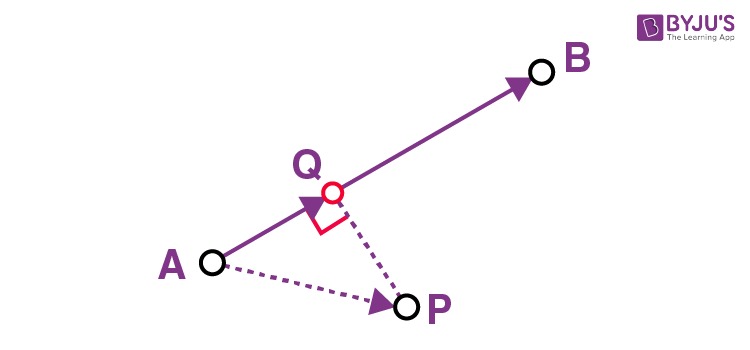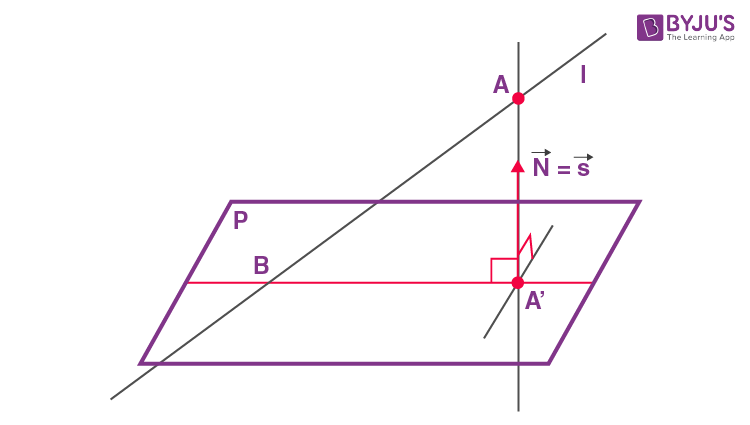# How to Find Projection of Line on Plane

3D geometry involves the study of shapes in 3D space and involves 3 coordinates, which are x-coordinate, y-coordinate and z-coordinate. In a 3d space, we need three parameters to find the exact location of a point. For JEE, three-dimensional geometry is very important as a lot of questions are included in the exam. In this article, we will learn how to find Projection of line on plane with an example.

## Projection of a Point on a Line

Consider line AB and a point P. Construct a perpendicular PQ from P on AB that meets AB at Q. This point Q is known as the projection of P on the line AB.## What is the Projection of a Line on a Plane?

The orthogonal projection of a line onto a plane is a line or a point. If a line is perpendicular to a plane, its projection is a point. The intersection point with the plane and its direction vector s will be coincident with the normal vector N of the plane.

If a line is parallel with a plane then it will be parallel with its projection onto the plane. It is orthogonal to the normal vector of the plane.

s⊥N

s.N = 0Projection of a line which is not parallel nor perpendicular to a plane will pass through their intersection B and through the projection A’ of any point A of the line onto the plane, as shown in the figure above.

### Example

Find the equations of the projection of the line (x+1)/-2 = (y-1)/3 = (z+2)/4 on the plane 2x+y+4z = 1.

Solution:

Given equation of line (x+1)/-2 = (y-1)/3 = (z+2)/4 = λ

So x = -2λ-1

y= 3λ+1

z= 4λ-2

Equation of plane is 2x+y+4z = 1

λ will satisfy the equation of the plane.

2(-2λ-1)+3λ+1+4(4λ-2) = 1

-4λ-2+3λ+1+16λ-8 = 1

15λ-10 = 0

15λ = 10

λ = 10/15 = ⅔

Substitute λ in x, y and z and find the corresponding values.

x = -2λ-1 = -2(⅔)-1 = -7/3

y = 3λ+1 = 3(⅔)+1 = 3

z= 4λ-2 = 4(⅔)-2 = ⅔

Coordinate of point B = (-7/3, 3, ⅔)

Coordinate of A = (-1,1,-2)

Equation of normal vector N = 2i+j+4k

Equation of line = (x-x0)/a = (y-y0)/b = (z-z0)/c

(x+1)/2 = (y-1)/1 = (z+2)/4 = t

So x = 2t-1

y= t+1

z= 4t-2

Substitute x, y, z in equation of plane

2(2t-1)+(t+1)+4(4t-2) = 1

21t = 10

t = 10/21

Substitute t in x, y, z

x = -1/21

y = 31/21

z = -2/21

Coordinate of point A’ = (-1/21, 31/21, -2/21)

Equation of line is (x-x1)/(x2-x1) = (y-y1)/(y2-y1) = (z-z1)/(z2-z1) ..(i)

(x1, y1, z1) = (-7/3, 3, ⅔)

(x2, y2, z2) = (-1/21, 31/21, -2/21)

Substitute (x1, y1, z1) and (x2, y2, z2) in (i)

(x+7/3)/((-1/21)+(7/3)) = (y-3)/((31/21)-3) = (z-(⅔))/((-2/21)-(⅔))

Solving we get (21x+49)/48 = 21(y-3)/-32 = (21z-14)/-16 which is the required equation.